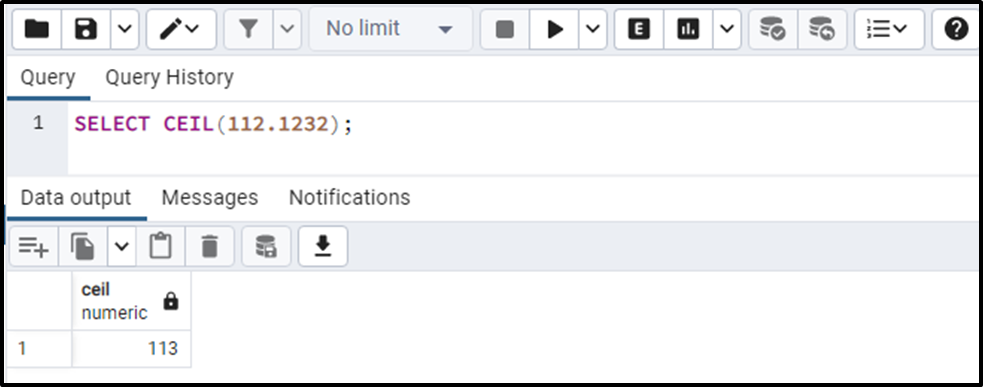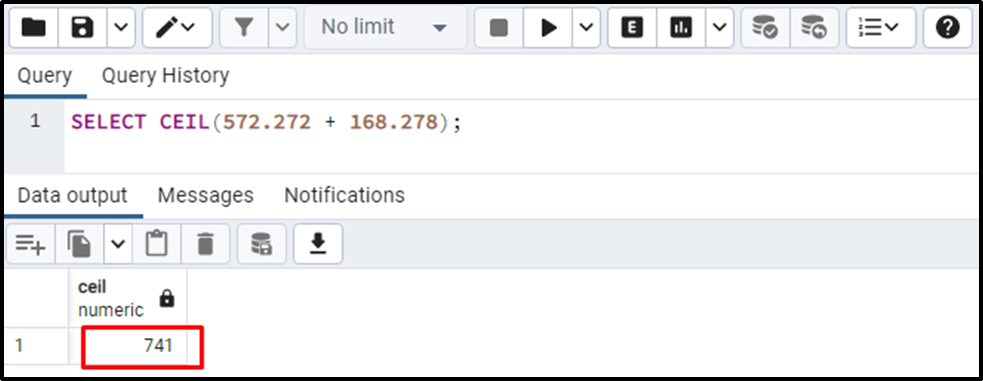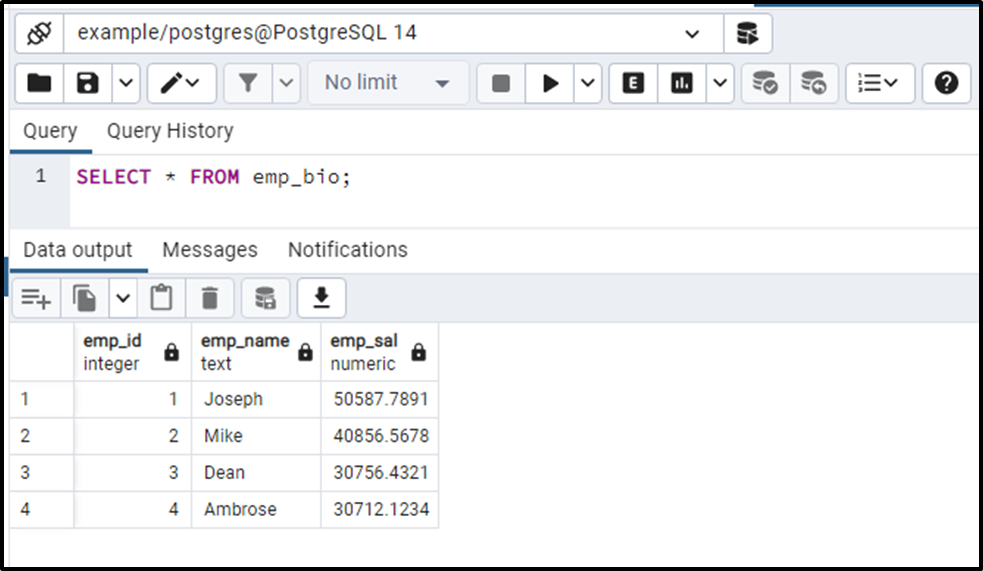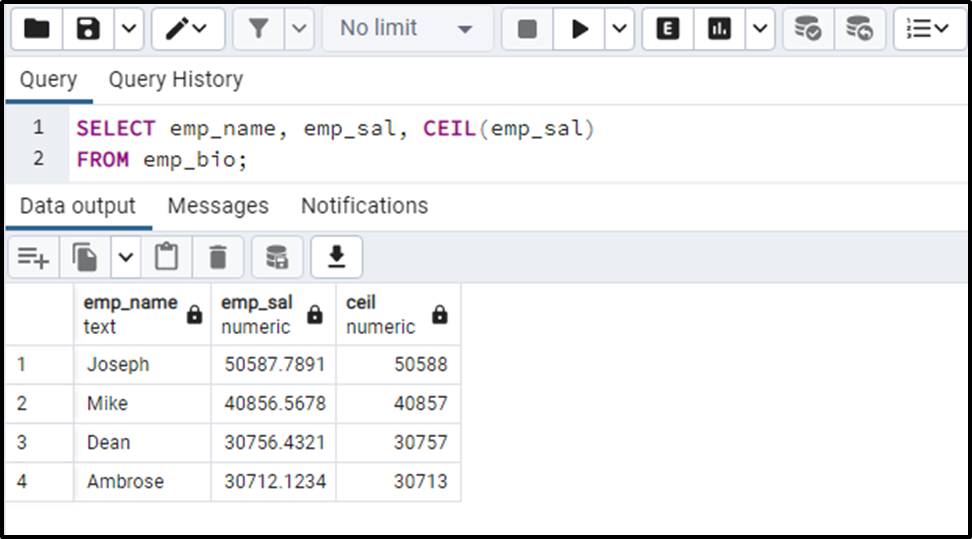##### How to Use CEIL() Function in PostgreSQL

In PostgreSQL, you can perform a variety of mathematical operations via built-in math functions, such as POWER(), SQRT(), etc. All these functions fulfill different tasks, for instance, finding the power of a number, the square root of a number, and so on. Similarly, PostgreSQL offers another convenient mathematical function named the CEIL() that rounds up a number to the next whole number.

This blog post will demonstrate the working of the CEIL() function via practical examples. So, let’s get started.

How to Use CEIL() Function in Postgres?

Postgres CEIL() function is one of the mathematical functions that take a numeric value or an expression as an argument and round up the given value/expression to the next whole number:

`CEIL(arg_1);`
• The above snippet shows that the CEIL() function accepts only a single argument, “arg_1”.
• The “arg_1” can be a numeric value or an expression.
• The retrieved value will have the same data type as the given numeric value/expression.

Example 1: How Does the CEIL() Function Work?

Let’s pass a numeric value to the CEIL() function and see how it works in Postgres:

`SELECT CEIL(112.1232);`

In the above snippet, the CEIL() function takes the number “112.1232”, and consequently, it retrieves the following output:The output shows that the CEIL() function rounded up the given number to the nearest integer.

Example 2: How Does the CEIL() Function Work With an Expression?

Let’s pass an expression instead of a number to the CEIL() function and see how it works:

`SELECT CEIL(572.272 + 168.278);`The output shows that the CEIL() function calculates the given expression and rounds the resultant value up to the nearest whole number.

Example 3: How to Use the CEIL() Function on Table’s Data?

We have already created an “emp_bio” table; let’s verify the selected table’s data via the SELECT command:

`SELECT * FROM emp_bio;`Now let’s utilize the CEIL() function on the emp_sal column of the emp_bio table:

```SELECT CEIL(emp_sal)
FROM emp_bio;```The output authenticates the working of the CEIL() function as it successfully rounded up the column’s values to the nearest integers.

Conclusion

Postgres provides a CEIL() function that takes a numeric value or an expression as an argument and rounds up the given value/expression to the next whole number. The retrieved value will have the same data type as the given numeric value/expression. This blog post presented a detailed guide on the CEIL() function via practical examples.